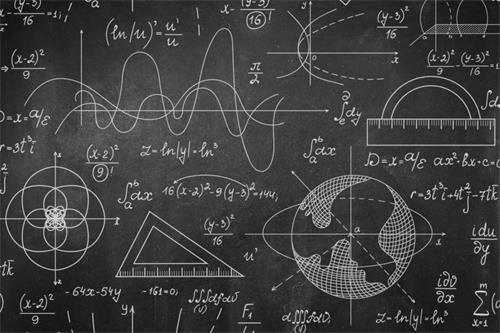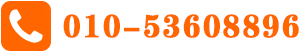# 2014年5/6月Alevel物理真题及答案解析

alevel真题及答案       时间：2019-08-19 15:31

今天A+教育小编为大家分享下2014年5/6月Alevel物理真题及答案解析，希望对各位准备Alevel的学生有所帮助。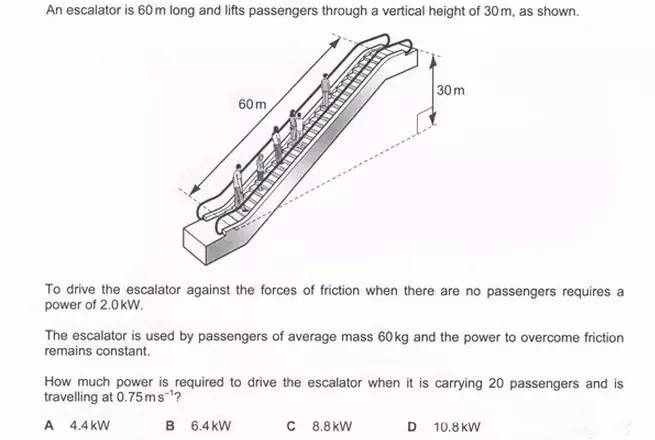答案：C

The power of passengers can be calculated by F*V=20*60*9.81*0.75=8.8kW. So the total power is 8.8kW.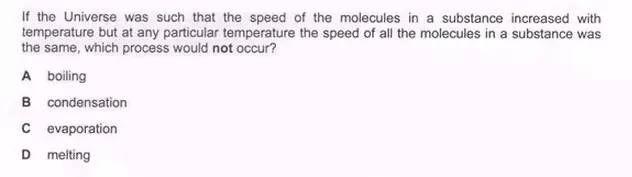答案：D

The temperature is constant when melting.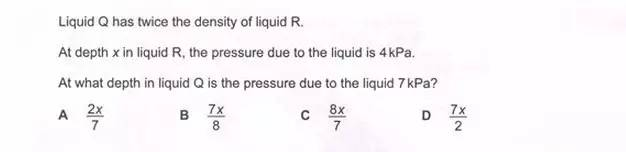答案：B

4=ρRgx, 7=ρQgy, ρQ=2ρR, so 7x/8.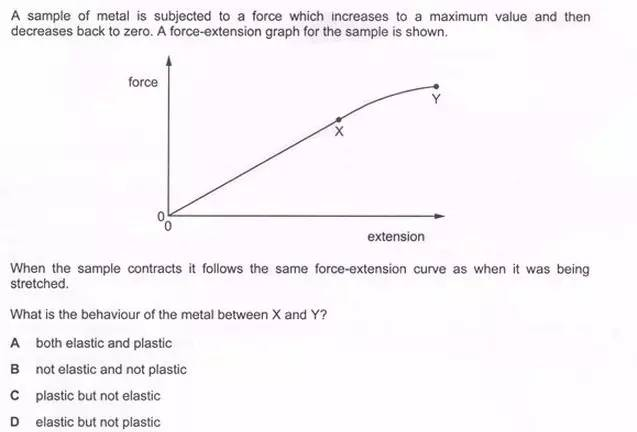答案：D

The metal can go back to the original point， so the metal is still in elastic statement.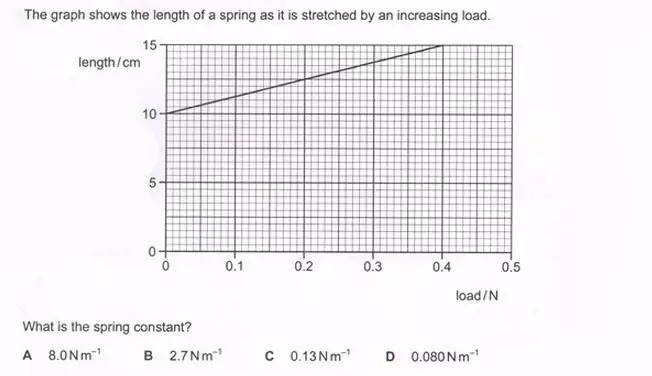答案：A

The gradient of extension-load graph is 1/spring constant. The gradient is 0.125, so the spring constant is 8N/m.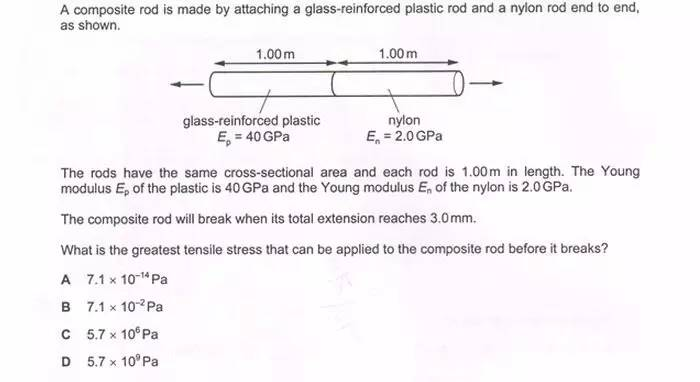答案：C

Total extension =strain*original length. Total strain=stress/E1+stress/E2. So the answer is 5.7*106Pa.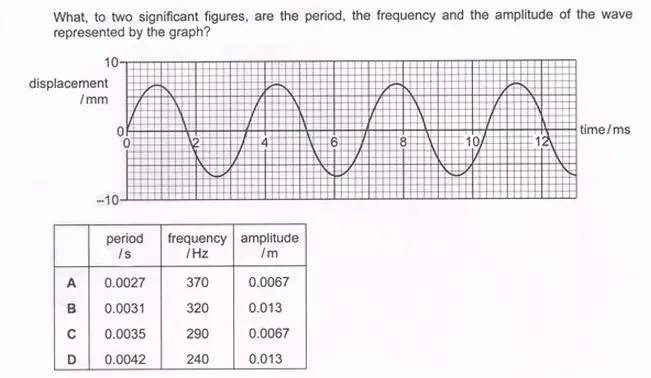答案：C

From the graph the period is 3.5ms， the amplitude is 6.7mm.答案：A

The resistance of the wire is 12/0.83=14.45Ω, the total length is 36m, so the answer is 0.04Ω.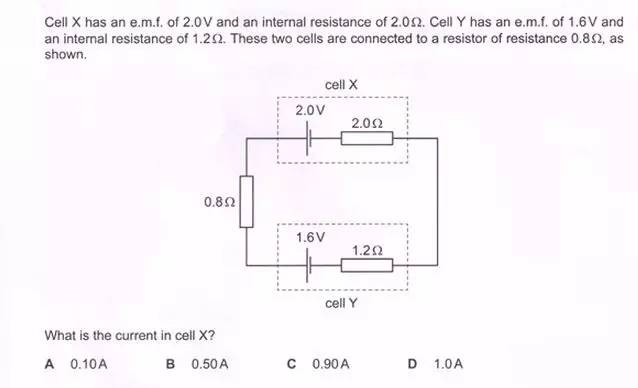答案：A

The total potential difference is 0.4V，the total resistance is 4Ω，so the current is 0.1A.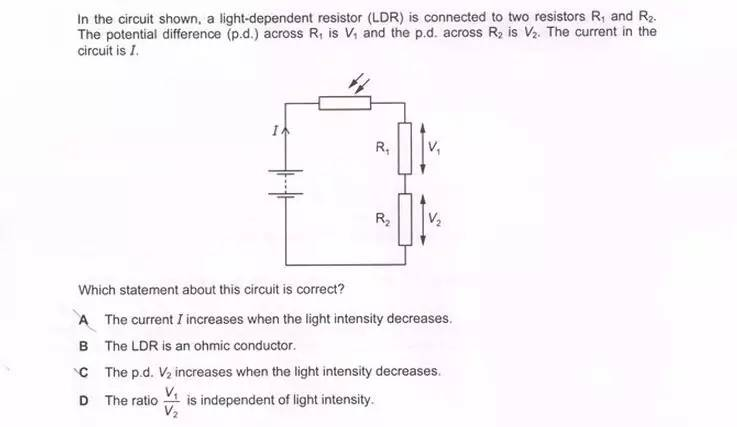答案：A

When the intensity is increased，the resistance of LDR will decrease，so the current will increase.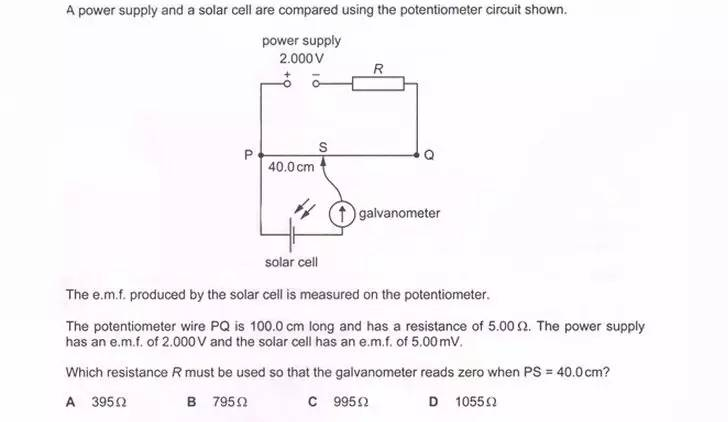答案：B

When the galvanometer is 0，the potential of PS is 5mV，so the total potential of PQ is 12.5mV. According to the potential is proportional to the resistance，the answer is 795.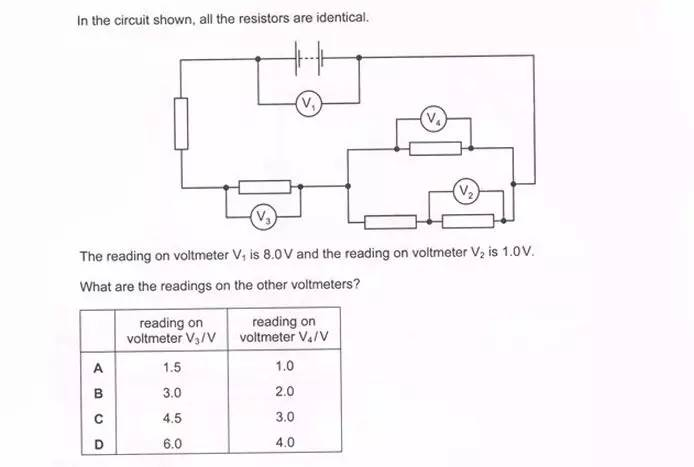答案：B

The potential of V4 is 2，the potential of V3 is 3.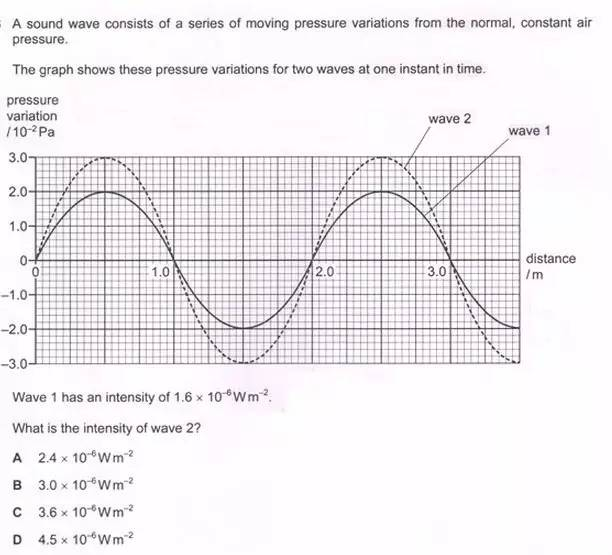答案：C

Intensity is directly proportional to the amplitude2&frequency2.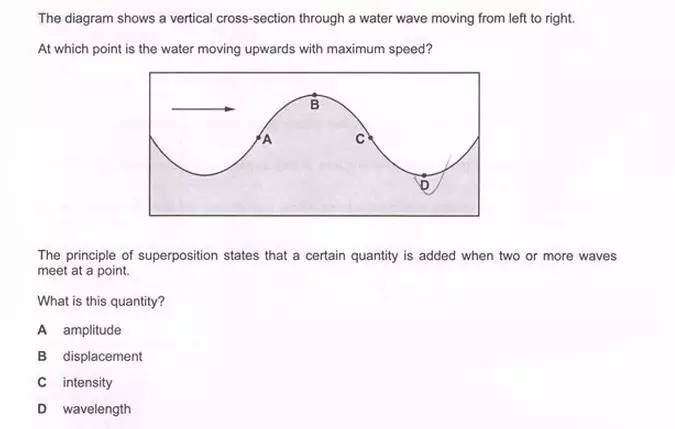答案：C

The particle is at its maximum velocity when it is in equilibrium position.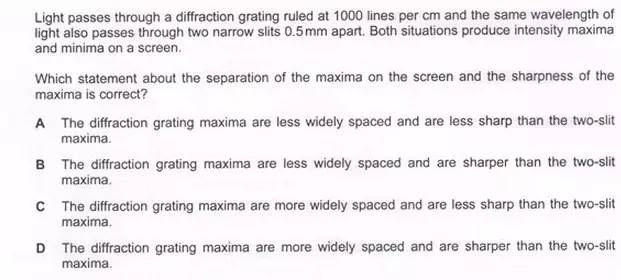答案：C

According to the corresponding formula，the wide length of diffraction is larger than double-slit interference，but the intensity is less.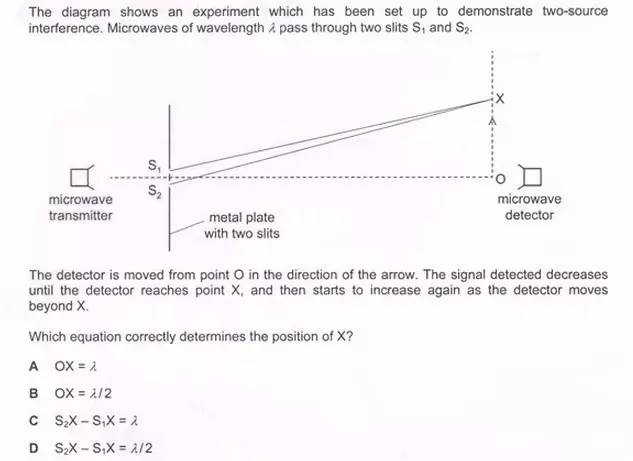答案：C

X is the bright pattern，so the path difference is λ.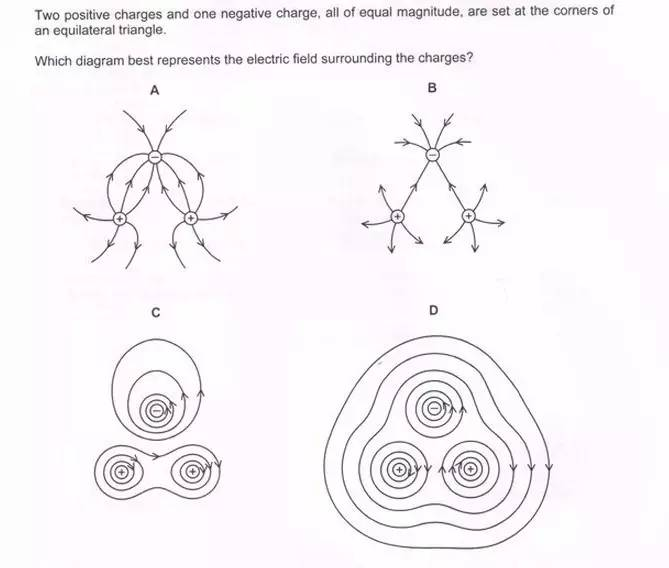答案：A

Learn it from the electric field of two same charged particles and repulsive particles.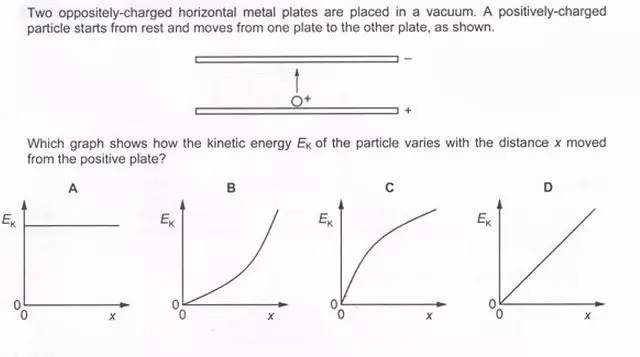答案：D

qEx=Ek, so Ek is proportional to the x.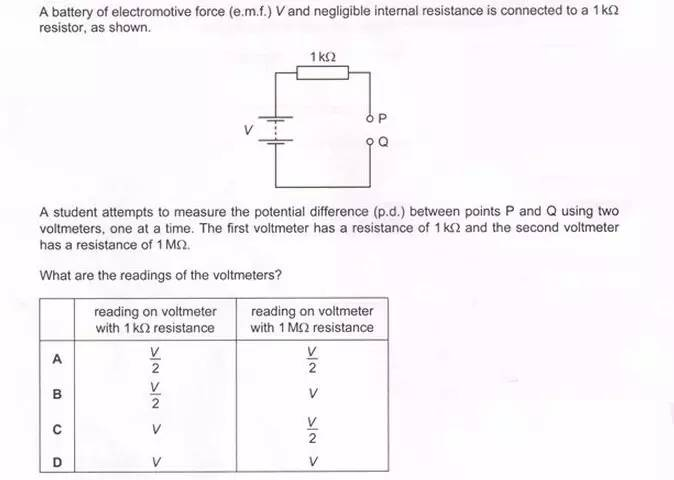答案： B

The potential difference is proportional to the resistance in series circuit.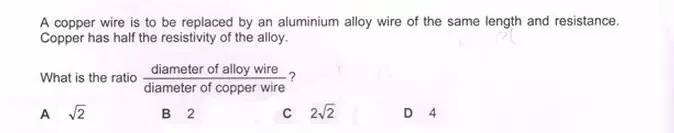答案：A

R=ρ1/A, A =πd2/4.

以上2014年5/6月Alevel物理真题及答案解析啦，如果各位同学哪里还有疑问，可随时与A+国际教育的老师进行一对一的免费咨询哦。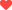喜欢 

## 最新文章 More## 相关推荐#### 2014年5/6月Alevel物理真题及答案解析

2014年5/6月Alevel物理真题及答案解析.....

## 猜你喜欢 More